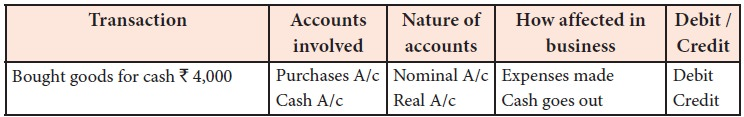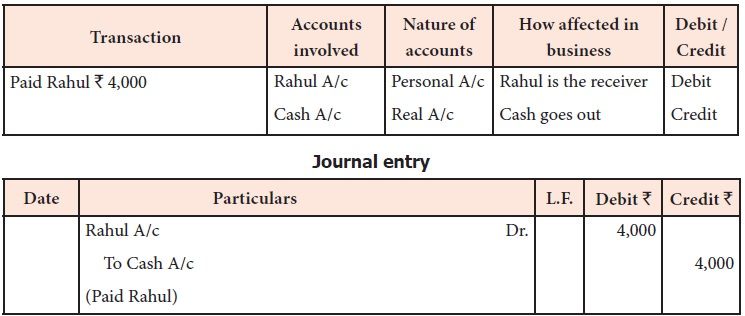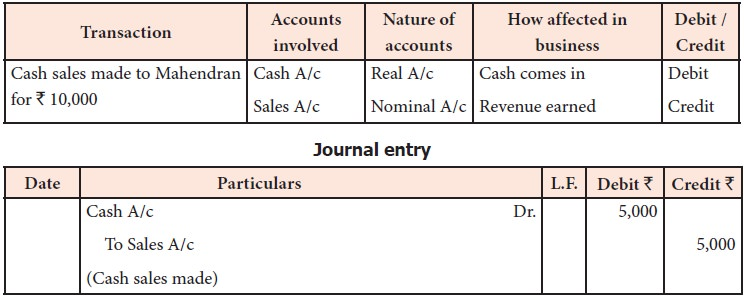Home | | Accountancy 11th std | Analysis of transactions with Example

# Analysis of transactions with Example

Accountancy : Books of Prime Entry - Analysis of transactions with Example

## Analysis of transactions

### Example 1

Transaction: Somu commenced printing business with cash Rs. 50,000.

Analysis: This is a cash transaction as cash is involved.### Example 2

Transaction: Bought goods for cash Rs. 4,000

Analysis: This is a cash transaction as cash is involved.### Example 3

Transaction: Purchased goods from Rahul for Rs. 10,000 on credit

Analysis: This is a credit transaction.### Example 4

Transaction: Cash paid to Rahul Rs. 4,000

Analysis: This is a cash transaction as cash is involved.### Example 5

Transaction: Withdrew cash for personal use Rs. 8,000

Analysis: This is a cash transaction as cash is involved.### Example 6

Transaction: Cash deposited into bank Rs. 5,000

Analysis: This is a cash transaction as cash is involved.### Example 7

Transaction: Paid salary by cheque Rs. 3,000

Analysis: This is a bank transaction as bank is involved.### Example 8

Transaction: Sold goods to Mahesh on credit Rs. 9,000

Analysis: This is a credit transaction.### Example 9

Transaction: Goods sold to Mahendran for cash Rs. 5,000

Analysis: This is a cash transaction as cash is involved.### Example 10

Transaction: Borrowed loan from Bank Rs. 10,000

Analysis: This is a cash transaction as cash is involved.### Example 11

Transaction: Received commission of Rs. 5,000 by cash

Analysis: This is a cash transaction as cash is involved.Example 12

Transaction: Paid Rs. 4,800 in full settlement of Rs. 5,000 due to the creditor, Keerthana.

Analysis: This is a cash transaction as cash is involved.### Example 13

Transaction: Dinesh, a customer is declared insolvent and 40 paise in a rupee is received from the estate for his due Rs. 10,000.

Analysis: This is a cash transaction as cash is involved.Tutorial note

Though the procedure for analysis of transactions, classification of accounts and rules for recording transactions under accounting equation approach and traditional approach are different, the accounts affected and entries in affected accounts remain the same under both approaches. In other words, accounts to be debited and credited to record the dual aspect remain the same under both the approaches.

Tags : Books of Prime Entry | Accountancy , 11th Accountancy : Chapter 3 : Books of Prime Entry
Study Material, Lecturing Notes, Assignment, Reference, Wiki description explanation, brief detail
11th Accountancy : Chapter 3 : Books of Prime Entry : Analysis of transactions with Example | Books of Prime Entry | Accountancy